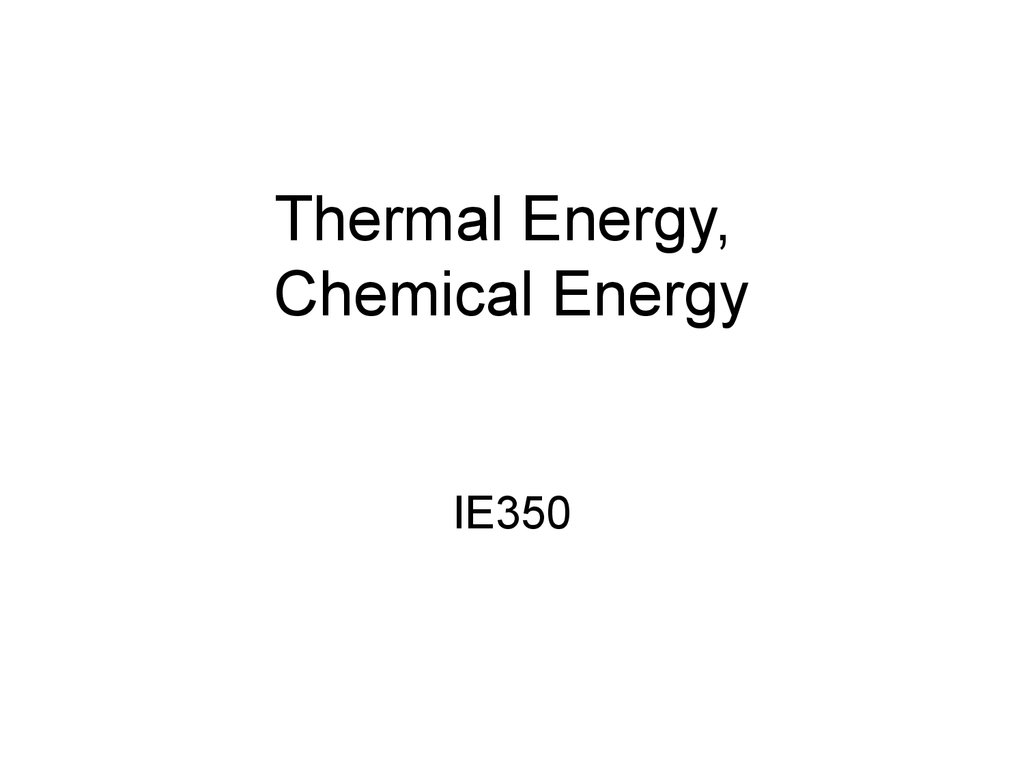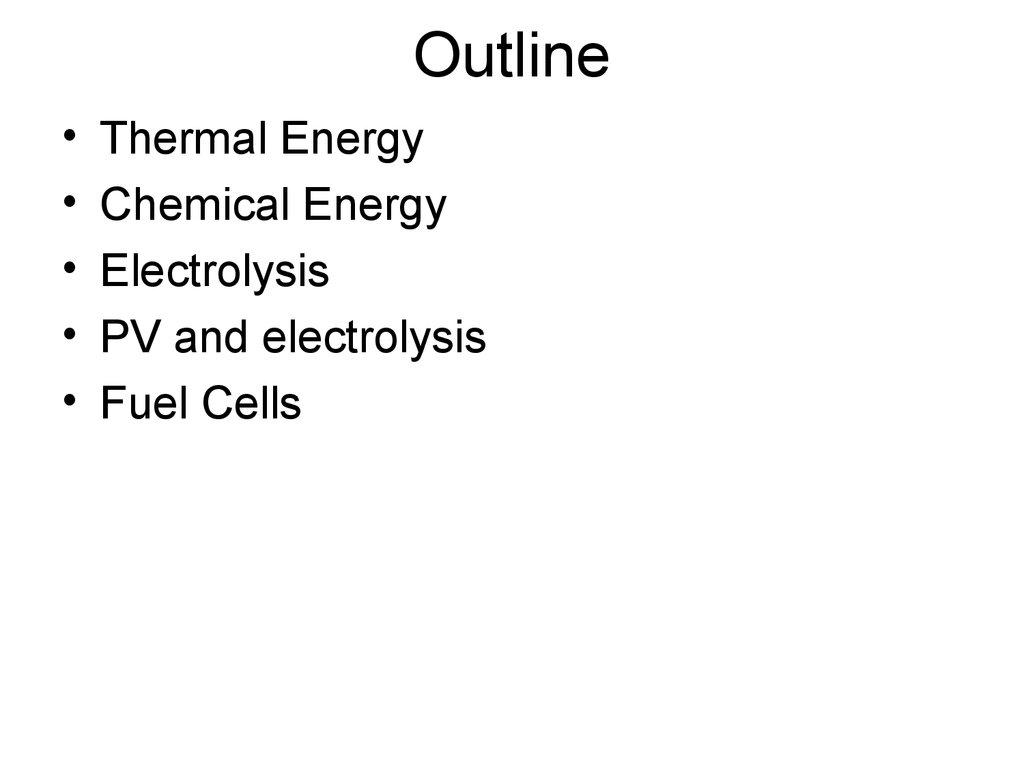Thermal Energy, Chemical Energy

IE350

2. Outline

Thermal Energy
Chemical Energy
Electrolysis
PV and electrolysis
Fuel Cells

3. Thermal Energy

• We already know that in order to increase
by 1°C the 1 gram of water we need 1
calorie.
• For any mass and any temperature
difference we will have:
Q = C·m· t,
where C is the Specific Heat

4. Specific Heat

• The Specific Heat measurement unit, c
naturally is:
cal/(g·°C) =
= 4.184 J/(g·°C) or J/(g·°K)
• Water has a mass-specific heat capacity
of about 4.184 joules per Kelvin per gram
near 20 °C.
• … or 1 calorie per kelvin per gram near
20 °C (this is again the calorie definition).

5. Heat Storage

• Assume you have 1 ton of water at 94°C in a
room. After some time the temperature
decreases to 24°C. How much energy is
released to the room?
Q = c·m· t
c = 4.184 MJ/(ton·°K)
m=1t
t = 70°C
Q = 4.184 · 70 MJ = 292.88 MJ = 81.35 kWh
(1 kWh = 3.6 MJ).

6. Table of Specific Heat for Various Materials.

Substance
Asphalt
Brick
Concrete
• Which material is best for Glass, pyrex
heat storage?
Granite
• Remember that water is
Gypsum
limited in t, e.g. bricks
or granite – not so much. Marble, mica
Sand
• However losses at larger
Soil
t-s are much higher.
Wood
Water
cp
J g−1 K−1
0.92
0.84
0.88
0.753
0.790
1.09
0.880
0.835
0.80
0.42
4.1813

• Water:
- gas,100 °C
- liquid, 25 °C
- solid, 0 °C
J/(g·°K)
2.08
4.1813
2.114

9. Losses

Losses are linearly
related to the
temperature difference
t (temperature

10. Specific Heat of: Fusion and Vaporization

• Specific Heat of Fusion:
Amount of energy needed to turn solid into
liquid.
• Specific Heat of Vaporization:
Amount of energy needed to turn liquid
into vapor.

11. H2O: From Ice to Vapor

1.
2.
3.
4.
5.
How much Energy is needed to turn ice into
vapor?
5 steps of calculation:
Energy needed to reach the melting point;
Add energy needed to melt the ice;
Add energy needed to reach the vaporization
point;
Add energy needed to vaporize the water;
Add energy needed to reach higher
temperature of vapor (analogy with band gap
in Si).

12. H2O: From Ice to Vapor

- Energy needed to melt the ice:
333 J/g = specific heat of fusion
- Energy needed to vaporize the water:
2260 J/g = specific heat of vaporization
- How this difference is explained?

17. Enthalpy

• Enthalpy or heat content (denoted as H or ΔH,
or rarely as χ) is a quotient or description of
thermodynamic potential of a system, which
can be used to calculate the "useful" work
obtainable from a closed thermodynamic
system under constant pressure,
• Short definition: Enthalpy is the energy density
in heat-mass transfer (transportation)
phenomena.

18. Enthalpy

• Enthalpy,
H = {Energy content}/mass = E/m
measured in J/g or J/kg.
• Importantly, in many cases H =
Q/m.

19. Humidity

• Absolute
• Relative
• Absolute Humidity = weight of
water in the volume of air,
g/m3;
• … or weight of water in weight
of air, g/kg.

20. Relative humidity

Relative humidity is defined as the ratio of the partial pressure (or
density) of water vapor in a gaseous mixture of air and water to the
saturated vapor pressure (or density) of water at a given
temperature. Relative humidity is expressed as a percentage and is
calculated in the following manner:
RH = 100% • [p(H2O)]/[p*(H2O)]
where:
RH is the relative humidity of the gas mixture being considered;
is the partial pressure of water vapor in the gas mixture; and
is the saturation vapor pressure of water at the temperature
of the gas mixture.

DRY BULB
WET BULB

25. Chemical Energy

• The weight of a proton or neutron is
1.66 · 10-24 g
• Since the electron weight is too small compared
to proton, 1/1837 –th, the weight of atoms is
defined by protons and neutrons.
• NA, Avogadro Number, = 6.022 ·1023mol-1
particles. The unit of amount of substance.
• number of atoms in 12g of the isotope carbon-12
• Interesting is that the volume of 1 mol of ideal
gas is always the same. Precisely,
22.414 (dm)3/mol at 0 °C
24.465 (dm)3/mol at 25 °C

• October 23 is called Mole Day. It is an
informal holiday in honor of the unit
among chemists. The date is derived
approximately 6.022×1023. It starts at
6:02 a.m. and ends at 6:02 p.m.

27. Heat of Formation

• Reactions can be endothermic –
absorption of heat takes place,
temperature of ambience is decreased;
• or exothermic – release of heat takes
place, temperature of ambience is
increased;
• Denoted by Hf° - amount of energy per
unit amount of substance, kcal/mol,
released or absorbed by a reaction – is
the reaction enthalpy.

29. Exothermic & Endothermic reactions

Exothermic & Endothermic reactions

30. Heats of Formation

Compound
Notation
Hf°, kcal/mol
Carbon dioxide
CO2
-94
Water
H2O
-68
Methane
CH4
-17.9
Gasoline
C8H18
-49.8
H2, O2, N2, F2, ..
0
Diatomic gases

31. Hydrogen and water

• We all know:
H 2 + O 2 H2O
• But the correct reaction formula is:
2H2 + O2 2H2O
• This is stoichiometric reaction, and the
result is 2 moles of water, thus with energy
balance the equation will be:
2H2 + O2 2H2O – 136 kcal,
since water Hf° = - 68 kcal/mol
= - 286 kJ/mol.

• However, what
is the future?
• Hydrogen
Combustion
Engines?
• Hydrogen
Fuel Cells?
• Large Ocean
Solar Stations?

33. PV and electrolysis.

• Storage of solar energy is a problem yet to
be solved.
• Hydrogen is one of the best solutions.
• Electrolysis efficiency is about 80%, with
theoretical maximum of 94%.
• Safety problems: The enthalpy of
combustion for hydrogen is 286 kJ/mol,
• Burning concentration starts from 4% (v)!
• However, as experience shows, it is safer
than e.g. gasoline or methane!

38. Fuel cells

Its efficiency is a
function primarily of
membrane and
electrocatalyst
performance.
This becomes
crucial under highcurrent operation,
which is necessary
for industrial-scale
application.

39. Photoelectrochemical cells

In this type of photoelectrochemical cells,
electrolysis of water to hydrogen and oxygen gas
occurs when the anode is irradiated with
suggested as a way of converting solar energy into
a transportable form, namely hydrogen. The
photogeneration cells passed the 10 percent
economic efficiency barrier.
Lab tests confirmed the efficiency of the process.
The main problem is the corrosion of the
semiconductors which are in direct contact with
water. Research is going on to meet the DOE
requirement, a service life of 10000 hours.
Photogeneration cells have passed the 10 percent
economic efficiency barrier. Corrosion of the
semiconductors remains an issue, given their direct
contact with water. Research is now ongoing to
reach a service life of 10000 hours, a requirement
established by the United States Department of
Energy

40. How to store Hydrogen?

Cylinders – compressed hydrogen
Metal Hydrate Compounds
Cryogenic storage
Chemical Storage
Carbon nanotube storage
Glass Microspheres
Liquid carrier storage

42. Cylinders – compressed hydrogen

• requires energy to
acomplish
• lower energy density
when compared to a
• same energy content
yields a tank that is
3,000 times bigger than
the gasoline tank

43. Metal Hydrates

• MgH2, NaAlH4, LiAlH4, LiH, LaNi5H6,
• similar to a sponge, 1-2% of the weight.
• could reach to 5-7% if heated to 250°C
• delivering Hydrogen at a constant
pressure.
• it also absorbs any impurities introduced
into the tank by the hydrogen. The result
is the hydrogen released from the tank
is extremely pure, but the tank's lifetime
and ability to store hydrogen is reduced
as the impurities are left behind and fill
the spaces in the metal that the
hydrogen once occupied.

44. Cryogenic storage

• Liquid hydrogen typically has to
be stored at 20o Kelvin or -253o C.
• again, necessitate spending
energy to compress and chill the
hydrogen into its liquid state,
resulting in a net loss of about
30% of the energy that the liquid
hydrogen is storing.
• a similar percentage will be due to
t is usually > 270°C!
• Larger, composite material tanks
would be beneficial.

46. Chemical Storage

• Some examples of various techniques
include ammonia cracking, partial
oxidation, methanol cracking, etc. These
methods eliminate the need for a storage
unit for the hydrogen produced, where the
hydrogen is produced on demand.
• Still in the research stage.

47. Carbon nanotube storage

• Carbon nanotubes
are microscopic tubes
of carbon, two
nanometers (billionths
of a meter) across,
that store hydrogen in
microscopic pores on
the tubes and within
the tube structures.
• 4.2% - to 65% of their
own weight in
hydrogen!
Carbon
nanotube
storage

48. Glass Microspheres

• Tiny hollow glass spheres can be
used to safely store hydrogen. The
glass spheres are warmed,
increasing the permeability of their
walls, and filled by being immersed
in high-pressure hydrogen gas.
• The spheres are then cooled,
locking the hydrogen inside of the
glass balls. A subsequent increase
in temperature will release the
hydrogen trapped in the spheres.
• Microspheres have the potential to
be very safe, resist contamination,
and contain hydrogen at a low
pressure increasing the margin of
safety.

49. Liquid Carrier (Carbohydrate) Storage

• This is the technical term for the hydrogen being
stored in the fossil fuels that are common in
today's society. Whenever gasoline, natural gas
methanol, etc.. is utilized as the source for
hydrogen, the fossil fuel requires reforming.
• The reforming process removes the hydrogen
from the original fossil fuel.
• The reformed hydrogen is then cleaned of
excess carbon monoxide, which can poison
certain types of fuel cells, and utilized by the fuel
cell.
• Reformers are currently in the beta stage of their
testing with many companies having operating
prototypes in the field.

50. Hydrogen Safety

• The range of explosion proportion in air is
rather wide, starting at 4%.
• Hydrogen is light – it goes up in
atmosphere.
• Hydrogen molecules are small – they
penetrate and escape from many
situtations.

51. Hydrogen Use

• Internal Combustion
Engines
• PEM Fuel Cells

53. PEM Fuel Cells

• Acts like a battery, delivering electricity
with efficiencies around 55%.
• This “battery” does not need to spend time
on recharging! Whenever H2 and O2 (or
humidified air) are supplied – it operates.
• The rest of the energy can theoretically be
used – in a form of heat.
• Excellent way to provide distributed power
and integrate with renewable sources.

H2ECOnomy

56. Homework

1. Assume that a household needs 3 kW
heating power on average of 24 hours
during any day, during the 4.5 months of
winter period. What kind of seasonal heat
storage you may suggest (material, size,
controllability, t, price)? Explain why and
make the calculation.
2. Calculate the heat content and the daily
amount of the hydrogen gas needed to
power the daily need to run a fuel cell
powered smartphone for 12 hours, 2.5W.
Assume conversion efficiency of 43%.